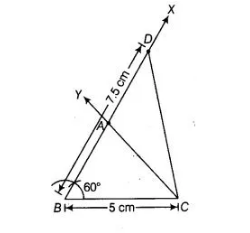# Construct a ΔABC in which BC = 5 cm,`
Question:

Construct a ΔABC in which BC = 5 cm, ∠B = 60° and

Solution:

Given, in $\triangle A B C, B C=5 \mathrm{~cm}, \angle B=60^{\circ}$ and $A C+A B=7.5 \mathrm{~cm}$. To construct the triangle ABC use the following steps.

1. Draw the base $B C=5 \mathrm{~cm}$.

2. At the point $B$ make an $\angle X B C=60^{\circ}$.

3. Cut a line segment $B D$ equal to $A B+A C=7.5 \mathrm{~cm}$ from the ray $B X$.4. Join DC.

5. Make an $\angle D C Y=\angle B D C$.

6. Let $\mathrm{CY}$ intersect $\mathrm{BX}$ at $\mathrm{A}$.

Then, $\triangle \mathrm{ABC}$ is the required triangle.